# Dividing Decimals - Definition with Examples

The Complete K-5 Math Learning Program Built for Your Child

• 30 Million Kids

Loved by kids and parent worldwide

• 50,000 Schools

Trusted by teachers across schools

• Comprehensive Curriculum

Aligned to Common Core

## Dividing Decimals

A decimal number has a whole number part and the fractional part separated by a dot. The dot in a decimal number is called a decimal point. The digits following the decimal point show a value smaller than one.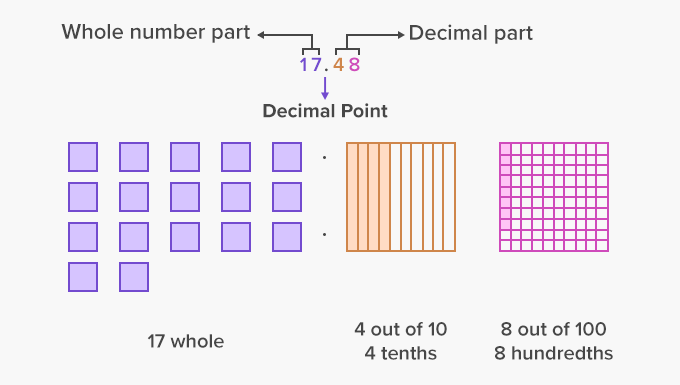Here's an example of a decimal number 17.48, in which 17 is the whole number, and 48 is the decimal part.

A. Dividing a decimal number by a whole number

Divide 120.6 by 24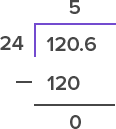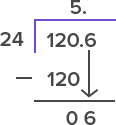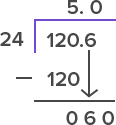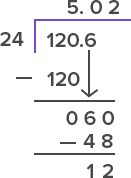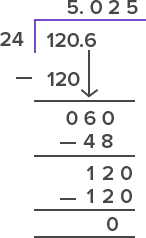Write the division in the standard form. Divide the whole number part of the decimal number by the divisor. Place the decimal point in the quotient above the decimal point of the dividend. Bring down the tenths digit. If the tenths digit cannot be divided by the divisor, write 0 in the quotient and in front of the tenths digit. Divide the dividend by the divisor. Keep adding the zeroes in the dividend until 0 is obtained in the remainder.

So, 120.6 ÷ 24 = 5.025

Example 2: Divide 230.5 by 5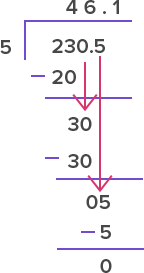So, 230.5 ÷ 5 = 46.1

B. Dividing a decimal number by another decimal number

Example: Divide 4.88 by 0.4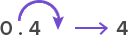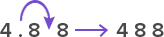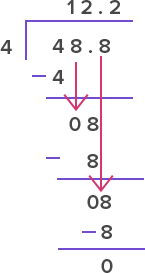Convert the divisor into a whole number by moving the decimal point to the right. Move the decimal point in the dividend the same number of places as in the divisor. Divide the decimal number by the whole number.

 Fun Facts In division, when remainder becomes 0 after a few decimal places in the quotient, it is called terminating decimals. Example, 62.75 ÷ 5 = 12.55. However, sometimes, the remainder never becomes zero and a digit or set of digits keep repeating in the quotient. This is called a recurring decimal. Example, 0.08 ÷ 0.3 = 0.2666666666….

Won Numerous Awards & Honors